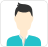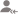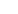Find the best tutors and institutes for Class 12 Tuition

Find Best Class 12 Tuition

Please select a Category.

Please select a Locality.

No matching category found.

No matching Locality found.

Outside India?

Search for topics# Class 12 Tuition Updates

Post a Lesson

Answered 6 days ago CBSE/Class 12/Mathematics/Vector Algebra/NCERT Solutions/Exercise 10.2

Find the values of x and y so that the vectors are equal read more

Find the values of x and y so that the vectorsare equal read lessShivani Sachdeva

The two vectors will be equal if their corresponding components are equal. Hence, the required values of x and y are 2 and 3 respectively. read more

The two vectorswill be equal if their corresponding components are equal.

Hence, the required values of x and y are 2 and 3 respectively.

Dislike Bookmark

Answered 6 days ago CBSE/Class 12/Mathematics/Vector Algebra/NCERT Solutions/Exercise 10.2

Find the scalar and vector components of the vector with initial point (2, 1) and terminal point (–5, 7). read more

Find the scalar and vector components of the vector with initial point (2, 1) and terminal point (–5, 7). read lessShivani Sachdeva

The vector with the initial point P (2, 1) and terminal point Q (–5, 7) can be given by, Hence, the required scalar components are –7 and 6 while the vector components are read more

The vector with the initial point P (2, 1) and terminal point Q (–5, 7) can be given by,Hence, the required scalar components are –7 and 6 while the vector components areDislike Bookmark

Answered 6 days ago CBSE/Class 12/Mathematics/Vector Algebra/NCERT Solutions/Exercise 10.2

Find the sum of the vectors. read more
Find the sum of the vectors. read lessShivani Sachdeva

The given vectors are. read more

The given vectors are.Dislike Bookmark

Looking for Class 12 Tuition

Find best Class 12 Tuition in your locality on UrbanPro.

FIND NOW

Answered 6 days ago CBSE/Class 12/Mathematics/Vector Algebra/NCERT Solutions/Exercise 10.2

Find the unit vector in the direction of the vector. read more
Find the unit vector in the direction of the vector. read lessShivani Sachdeva

The unit vector in the direction of vector is given by. read more

The unit vectorin the direction of vectoris given by.Dislike Bookmark

Answered 6 days ago CBSE/Class 12/Mathematics/Vector Algebra/NCERT Solutions/Exercise 10.2

Find the unit vector in the direction of vector, where P and Q are the points (1, 2, 3) and (4, 5, 6), respectively. read more

Find the unit vector in the direction of vector, where P and Q are the points

(1, 2, 3) and (4, 5, 6), respectively. read lessShivani Sachdeva

The given points are P (1, 2, 3) and Q (4, 5, 6). Hence, the unit vector in the direction of is . read more

The given points are P (1, 2, 3) and Q (4, 5, 6).Hence, the unit vector in the direction ofis.

Dislike Bookmark

Answered 6 days ago CBSE/Class 12/Mathematics/Vector Algebra/NCERT Solutions/Exercise 10.2Shivani Sachdeva

The given vectors are and. Hence, the unit vector in the direction of is(a→+b→)???a→+b→???=iˆ+kˆ2√=12√i?+12√k?.a→+b→a→+b→=i^+k^2=12i?+12k?. read more

The given vectors areand.Hence, the unit vector in the direction ofis
(a+b)???a+b???=iˆ+kˆ2=12i?+12k?.a→+b→a→+b→=i^+k^2=12i?+12k?.

Dislike Bookmark

Answered 6 days ago CBSE/Class 12/Mathematics/Vector Algebra/NCERT Solutions/Exercise 10.2

Find the position vector of a point R which divides the line joining two points P and Q whose position... read more

Find the position vector of a point R which divides the line joining two points P and Q whose position vectors arerespectively, in the ration 2:1

(i) internally

(ii) externally read lessShivani Sachdeva

The position vector of point R dividing the line segment joining two points P and Q in the ratio m: n is given by: Internally: Externally: Position vectors of P and Q are given as: (i) The position vector of point R which divides the line joining two points P and Q internally in the ratio... read more

The position vector of point R dividing the line segment joining two points

P and Q in the ratio m: n is given by:

1. Internally:1. Externally:Position vectors of P and Q are given as:(i) The position vector of point R which divides the line joining two points P and Q internally in the ratio 2:1 is given by,(ii) The position vector of point R which divides the line joining two points P and Q externally in the ratio 2:1 is given by,Dislike Bookmark

Answered 6 days ago CBSE/Class 12/Mathematics/Vector Algebra/NCERT Solutions/Exercise 10.2

Find the position vector of the mid point of the vector joining the points P (2, 3, 4) and Q (4, 1, – 2). read more

Find the position vector of the mid point of the vector joining the points P (2, 3, 4) and Q (4, 1, – 2). read lessShivani Sachdeva

The position vector of mid-point R of the vector joining points P (2, 3, 4) and Q (4, 1, – 2) is given by, read more

The position vector of mid-point R of the vector joining points P (2, 3, 4) and Q (4, 1, – 2) is given by,Dislike Bookmark

Answered 6 days ago CBSE/Class 12/Mathematics/Vector Algebra/NCERT Solutions/Exercise 10.2

Show that the points A, B and C with position vectors,, respectively form the vertices of a right angled triangle. read more

Show that the points A, B and C with position vectors,respectively form the vertices of a right angled triangle. read lessShivani Sachdeva

Position vectors of points A, B, and C are respectively given as:???AB−→−???2+???CA−→−???2=35+6=41=???BC−→−???2AB→2+CA→2=35+6=41=BC→2 Hence, ABC is a right-angled triangle. read more

Position vectors of points A, B, and C are respectively given as:???AB−→−???2+???CA−→−???2=35+6=41=???BC−→−???2AB→2+CA→2=35+6=41=BC→2

Hence, ABC is a right-angled triangle.

Dislike Bookmark

Looking for Class 12 Tuition

Find best Class 12 Tuition in your locality on UrbanPro.

FIND NOW

Answered 6 days ago CBSE/Class 12/Mathematics/Vector Algebra/NCERT Solutions/Exercise 10.2

In triangle ABC which of the following is not true: A. B. C. D. read more

In triangle ABC which of the following is not true:A.B.C.D.read lessShivani Sachdeva

On applying the triangle law of addition in the given triangle, we have: From equations (1) and (3), we have: Hence, the equation given in alternative C is incorrect. The correct answer is C. read moreOn applying the triangle law of addition in the given triangle, we have:From equations (1) and (3), we have:Hence, the equation given in alternative C is incorrect.

The correct answer is C.

Dislike Bookmark

UrbanPro.com helps you to connect with the best Class 12 Tuition in India. Post Your Requirement today and get connected.

Overview

Questions 19

Total Shares8 Followers

x